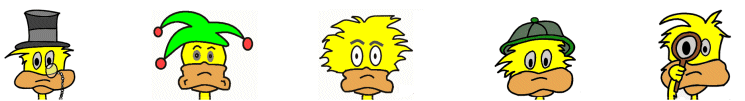# Kids Math

## Glossary and Terms: Units of Measurement

Length and Distance

Inch - The inch (or inches for plural) is a small unit of length. Twelve inches make up a foot. Compared to the metric system an inch equals 2.54 centimeters. Inches are used for smaller lengths such as measuring the length of a pencil or the width of an eraser.

Foot - A foot is a unit of length. There are 12 inches in a foot and 3 feet in a yard. Compared to the metric system a foot equals 0.3048 meters. Feet are used to measure medium size lengths such as the height of a person or the width of a room.

Yard - The yard is a unit of length used for medium size distances like the length of a football field. There are 3 feet in a yard. In the metric system 1 yard is 0.9144 meters.

Mile - The mile is used for long lengths like the distance between two cities. There are 5,280 feet in a mile. There are around 1.6 kilometers in a mile.

Meter - The metric system uses the meter as its basic unit of length. For very small lengths a millimeter is used which is 1000th of a meter.

Kilometer - The kilometer is used for long distances in the metric system. There are 1000 meters in a kilometer.

Volume

Fluid ounce - The fluid ounce is used to measure volume. There is also a unit called the ounce which is used for measuring mass. In the US, there are 8 fluid ounces in a cup. There are 12 fluid ounces in your average can of soda.

Cup - A cup is a medium sized unit of measure often used for measuring cooking ingredients. The US cup is defined as half a US pint. There are 8 cups in a quart and 16 cups in a gallon. There is also a metric cup. One US cup equals 0.946 metric cups.

Pint - A pint is another medium size unit of measure for volume. There are 2 cups in a pint.

Quart - A quart is used for slightly larger items. There are 4 cups in a quart and 4 quarts in a gallon.

Gallon - Gallons are used for measuring larger volumes like gas for your car. There are 4 quarts and 16 cups in a gallon. There are 3.78541 liters in a gallon.

Liter - The liter is the basic unit of volume for the metric system. There are 3.78541 liters in a gallon. For small volumes the milliliter is used. There are 1000 milliliters in a liter.

Weight and Mass

Ounce - The ounce is used for measuring small quantities of mass. Compared to the metric system, there are 28.3459 grams in a US ounce. It is abbreviated "oz." There are 16 ounces in a pound.

Pound - The pound is used for measuring the mass of medium to large objects such as a person. It is often abbreviated as lb. or lbs. for plural.

Ton - The ton is used for really large objects. A ton in the United States is 2,000 pounds. There is also the metric tonne (spelled differently) that equals 1,000 kilograms.

Gram - The gram is the basic metric unit of measurement for mass. For small measurements the milligram is used and for large measurements the kilogram is used. There are 28.3459 grams in a US ounce

Time

Second - The second is the smallest standard unit of time. Smaller units of time are based on the second such as the nanosecond or the microsecond. There are 60 seconds in a minute. Seconds are used to measure events of short duration like the 100 meter dash.

Minute - The minute is a measurement of time. There are 60 seconds in a minute and 60 minutes in an hour.

Hour - The hour is a medium length unit of time. There are 60 minutes in an hour and 24 hours in a day. Hours are used to measure durations such as the length of the school day or how long you sleep at night.

Day/Week/Month/Year - For longer durations we can use days, weeks, months, and years.

Temperature

Degrees - Degrees are used to measure the temperature or the hotness or coldness of an object. There are two main standard scales of degrees used including the Fahrenheit scale and the Celsius scale.

More Math Glossaries and Terms

Algebra glossary
Angles glossary
Figures and Shapes glossary
Fractions glossary
Graphs and lines glossary
Measurements glossary
Mathematical operations glossary
Probability and statistics glossary
Types of numbers glossary
Units of measurements glossary

Back to Kids Math

Back to Kids Study